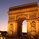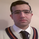14178 views
14178
Constance Brown's Derivative Oscillator was published in her book "Technical Analysis for the Trading Professional".

The oscillator uses a 14-period RSI . The RSI is then double smoothed with exponential moving averages. The default settings for the smoothing periods are 5 and 3.

In a second step a signal line is generated from the smoothed RSI by calculating a simple moving average with a period of 9.

The Derivative Oscillator is calculated as the difference between the smoothed RSI and the signal line and displayed as histogram.

All the values are configurable.
```//
// @author LazyBear
// @credits Constance Brown
//
study(title = "Derivative Oscillator [LazyBear]", shorttitle="DO_LB")
length=input(14, title="RSI Length")
p=input(9,title="SMA length")
ema1=input(5,title="EMA1 length")
ema2=input(3,title="EMA2 length")

s1=ema(ema(rsi(close, length), ema1),ema2)
s2=s1 - sma(s1,p)
c_color=s2 < 0 ? (s2 < s2 ? red : lime) : (s2 >= 0 ? (s2 > s2 ? lime : red) : na)
plot(s2 , style=histogram, color=c_color)
```Please pardon the newbie question, but is there a way to import the derivative oscillator into Thinkorswim's Thinkscript?Thanks for coding a broad range of indicators. Is this Indicator, what she refers as Composite index indicator?HamedAghajani
No. Composite index is available here -